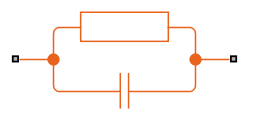# Foster Thermal Model

Heat transfer through a semiconductor module

•Libraries:
Simscape / Electrical / Passive / Thermal

## Description

Note

The Foster Thermal Model block represents an end-to-end behavioral model for temperature drop across the Tj and Tc ports. This block does not predict the heatflow rate at port B. You cannot extend this model with additional blocks either. To predict the heatflow rate at port B and to extend the model with additional blocks, use the Cauer Thermal Model block and select the Parameterize Cauer model using Foster coefficient data parameter.

The Foster Thermal Model block represents heat transfer through a semiconductor module. The figure shows an equivalent circuit for a fourth-order Foster Thermal Model block. Tj is the junction temperature and Tc is the base plate or ambient temperature.A Foster thermal model contains one or more instances of Foster thermal model elements. The figure shows an equivalent circuit for a Foster thermal model element.The number of thermal elements is equal to the order of representation. For a first order model, use scalar block parameters. For an nth order model, use row vectors of length n. Other terms that describe a Foster thermal model are:

• Partial fraction circuit

• Pi model

The defining equations for a first-order Foster thermal model element are:

`${C}_{thermal}=\frac{\tau }{{R}_{thermal}}$`

and

`${Q}_{AB}=\frac{{T}_{AB}}{{R}_{thermal}}+{C}_{thermal}\frac{d{T}_{AB}}{dt},$`

where:

• Cthermal is the thermal capacity.

• τ is the thermal time constant.

• Rthermal is the thermal resistance.

• QAB is the heat flow through the material.

• TAB is the temperature difference between the material layers.

### Initial Conditions

You can initialize the model in steady state or specify the initial node temperatures. To initialize the block in steady state, set the Start from steady state parameter to `On`. To specify the initial node temperatures and enable the Initial Targets section, set Start from steady state to `Off`. The first node temperature corresponds to the temperature in port A.

### Variables

To set the priority and initial target values for the block variables prior to simulation, use the Initial Targets section in the block dialog box or Property Inspector. For more information, see Set Priority and Initial Target for Block Variables.

Nominal values provide a way to specify the expected magnitude of a variable in a model. Using system scaling based on nominal values increases the simulation robustness. Nominal values can come from different sources, one of which is the Nominal Values section in the block dialog box or Property Inspector. For more information, see System Scaling by Nominal Values.

## Ports

### Conserving

expand all

Thermal conserving port associated with the left junction.

Thermal conserving port associated with the right junction.

## Parameters

expand all

Thermal resistance values, Rthermal , of the semiconductor module, specified as a vector.

Thermal time constant values, τ, of the semiconductor module, specified as a vector.

Whether to initialize the block in steady state.

 Schütze, T. AN2008-03: Thermal equivalent circuit models. Application Note. V1.0. Germany: Infineon Technologies AG, 2008.